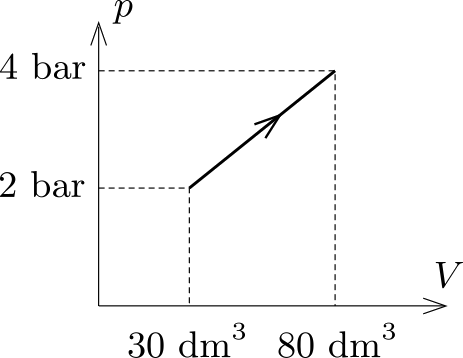Mathematical and Physical Journal
for High Schools
Issued by the MATFUND Foundation
 Already signed up? New to KöMaL?

#Problem P. 5051. (September 2018)

P. 5051. A sample of helium is taken through the processes shown in the figure. How much thermal energy is absorbed during the process?(4 pont)

Deadline expired on October 10, 2018.

Sorry, the solution is available only in Hungarian. Google translation

Megoldás. A belső energia megváltozása: $\displaystyle \Delta E=\frac{3}{2}p_2V_2-\frac{3}{2}p_1V_1=39~$kJ.

A gáz által végzett munka (a $\displaystyle p-V$ diagramon látható trapéz területe): $\displaystyle W'=15~$kJ.

A folyamat során felvett hő: $\displaystyle Q=\Delta E+W'=54~$kJ.

### Statistics:

 93 students sent a solution. 4 points: 53 students. 3 points: 16 students. 2 points: 8 students. 1 point: 7 students. 0 point: 8 students. Not shown because of missing birth date or parental permission: 1 solutions.

Problems in Physics of KöMaL, September 2018